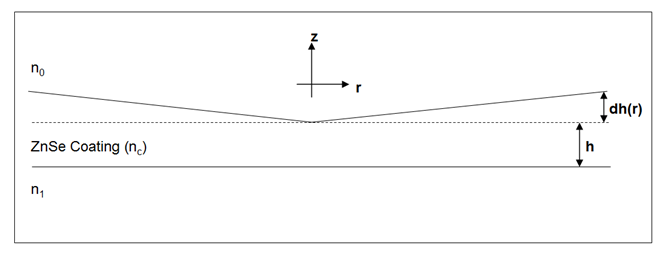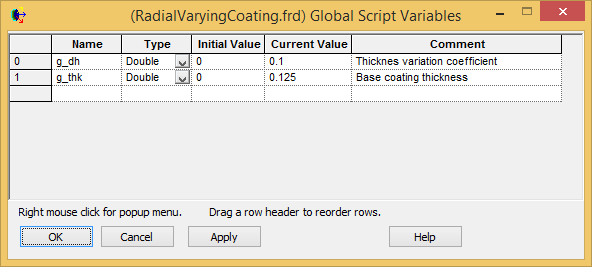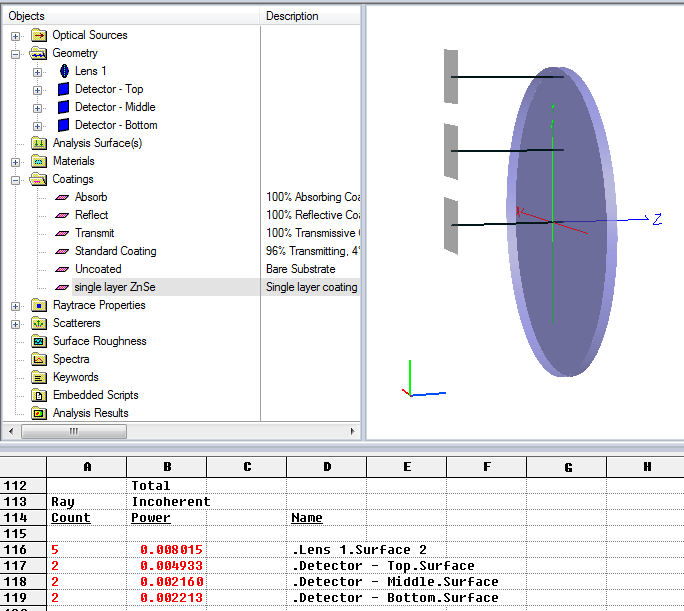Coatings can be applied to any surface in FRED using many different options. For specific solutions, the user can use the Script Coating option which allows reflection and transmission coefficients to be computed based on ray position, polarization, angle of incidence, wavelength and the complex index of refraction of the incident and substrate materials.

In this example, the Script Coating is used to create a single layer ZnSe (zinc selenide) coating with a thickness that increases linearly away from the center and is written to rigorously take into account polarization dependence and phase.The variables that can be used in the script are listed at the top of the Script Coating window when it is first opened, as shown below. This tells the user that the performance of the coating can be functions of the coordinates and angle of the incident ray, the refractive indices of the incident, and substrate materials and more.

```'Input  vars:  (g_x, g_y, g_z) = the point (x,y,z).
'              g_cos = cosine of the angle of incidence.
'              g_w = wavelength in microns.
'              g_n0 = refractive index of the incident material.
'              g_n1 = refractive index of the substrate material.
'              g_k0 = imaginary index of the incident material.
'              g_k1 = imaginary index of the substrate material.
'              g_refl = True --> compute reflection coefficients.
'                     = False --> compute transmission coefficients.<br>
```

The two user-defined parameters - the coating thickness (h) and the thickness variation coefficient (dh(r)) - are defined by the Global Variables g_thk and g_dh. These are accessed by selecting Tools -> Edit/View Global Script Variables… from the FRED main menu. Once defined here, they are accessible in any script associated with the FRED Document.The script must return the variables listed as Output vars. In this case, these are the magnitudes and phases of the s and p polarizations. Note that these output variables do not distinguish whether or not these are reflection or transmission coefficients. This is because each time the script runs, FRED obtains either the reflection value or transmission value, never both. This is flagged by the input variable g_refl. The script must be written to provide reflection coefficients if g_refl = True, otherwise provide transmission coefficients.

```'Output vars:  g_magS = magnitude of S-state coefficient.
'              g_magP = magnitude of P-state coefficient.
'              g_phsS = phase (radians) of S-state coefficient.
'              g_phsP = phase (radians) of P-state coefficient.<br>
```

At the top of the script, the refractive index of the ZnSe coating material is retrieved at the wavelength of interest, and the subsequent angles of refraction are calculated.

```    'Find layer material and refractive index at wavelength g_w
idm = FindMaterial( "ZnSe" )
nc = RefractiveIndex( idm, g_w )

'Angle of incidence
aoi = Acos(g_cos)

'Snells Law
ang2 = Asin( (g_n0/nc) * Sin(aoi) )
ang3 = Asin( (nc/g_n1) * Sin(ang2) )<br>
```

Next, the polarization-specific amplitude coefficients are calculated for both transmission and reflection at both the first interface (n0 / nc) and the second interface (nc / n1) using the well known Fresnel formulas. For example, the s-polarized (or “TE”) reflection from the first interface is defined by:

R(TE) = (s1 – s2)/ (s1 + s2)

where S1 = n0*cos(theta_0) and S2 = n1*cos(theta_c)

```    'TE (s polarization)
s1 = g_n0 * g_cos
s2 =   nc * Cos(ang2)
s3 = g_n1 * Cos(ang3)

'TM (p polarization)
p1 =     g_cos / g_n0
p2 = Cos(ang2) / nc
p3 = Cos(ang3) / g_n1

'1st interface
rTE12 = (s1-s2) / (s1+s2)
rTM12 = (p1-p2) / (p1+p2)
tTE12 =    2*s1 / (s1+s2)
tTM12 =    2*p1 / (p1+p2)

'2nd interface
rTE23 = (s2-s3) / (s2+s3)
rTM23 = (p2-p3) / (p2+p3)
tTE23 =    2*s2 / (s2+s3)
tTM23 =    2*p2 / (p2+p3)<br>
```

Next, using the Global Variables g_thk and g_dh, the height of the coating at the point of incidence is calculated and subsequently used to determine the phase shifts of the rays that propagate through the coating.

```    'Thickness variation using global script variables g_thk and g_dh
r = Sqr(g_x*g_x + g_y*g_y)
h = g_thk + (g_dh * r)

'Beta
b = (2*PI()/g_w) * nc * h * Cos(ang2)
If b > PI() Then b = b - PI()<br>
```

Then, the magnitude of the reflected and transmitted coefficients are calculated and returned in the output variables g_magS and g_magP.

```    'demoninators to be used for following calculations
denomS = 1 + 2*rTE12*rTE23*Cos(2*b) + rTE12^2*rTE23^2
denomP = 1 + 2*rTM12*rTM23*Cos(2*b) + rTM12^2*rTM23^2

'If computing reflection....
If g_refl Then

numSR = rTE12*(1+rTE23^2) + rTE23*(1+rTE12^2)*Cos(2*b)
numSI = rTE23*(1-rTE12^2) * Sin(2*b)
numPR = rTM12*(1+rTM23^2) + rTM23*(1+rTM12^2)*Cos(2*b)
numPI = rTM23*(1-rTM12^2) * Sin(2*b)

g_magS = Sqr(numSR^2+numSI^2) / denomS
g_magP = Sqr(numPR^2+numPI^2) / denomP

'If computing transmission....
Else

numSR = tTE12*tTE23*(1+rTE12*rTE23)*Cos(b)
numSI = tTE12*tTE23*(1-rTE12*rTE23)*Sin(b)
numPR = tTM12*tTM23*(1+rTM12*rTM23)*Cos(b)
numPI = tTM12*tTM23*(1-rTM12*rTM23)*Sin(b)

g_magS = Sqr(p3/p1)*Sqr(numSR^2+numSI^2) / denomS
g_magP = Sqr(q3/q1)*Sqr(numPR^2+numPI^2) / denomP

End If<br>
```

Finally, the phase of each polarization state is determined and assigned to the output variables g_phsS and gphsP.

```    'Calculate the phases for the S and P components
If numSR=0 Then
g_phsS=0
Else
g_phsS=Atn((numSI)/(numSR))
End If

If numPR=0 Then
g_phsP=0
Else
g_phsP=Atn((numPI)/(numPR))
End If<br>
```

Testing the Coating

The associated FRED Document provides a simple test of the coating implementation. Performing the raytrace and looking at the Ray Summary (Analyses / Ray Summary) shows that the reflected power from the coating that ends up on the three surfaces varies with the distance from the center.It should be noted, however, that the value for the angle of incidence of the incoming ray does not take into account the slope of the coating surface and therefore the assumption is that dh(r) << r.